# Coalescent Simulation with msprime

• 24k Accesses

Part of the Methods in Molecular Biology book series (MIMB,volume 2090)

## Abstract

Coalescent simulation is a fundamental tool in modern population genetics. The msprime library provides unprecedented scalability in terms of both the simulations that can be performed and the efficiency with which the results can be processed. We show how coalescent models for population structure and demography can be constructed using a simple Python API, as well as how we can process the results of such simulations to efficiently calculate statistics of interest. We illustrate msprime’s flexibility by implementing a simple (but functional) approximate Bayesian computation inference method in just a few tens of lines of code.

### Key words

• Population genetics
• Coalescent theory
• Simulation
• Python

Springer Nature is developing a new tool to find and evaluate Protocols. Learn more

## 1 Introduction

Thanks to the rapid advances in sequencing technology, generating genome-wide sequence datasets for many species has become routine and there is great interest in learning about the history of populations from sequence variation. The coalescent [15, 25, 40] gives an elegant mathematical description of the ancestry of a sample of sequences from a more or less idealized population and, given its focus on samples, has become the backbone of modern population genetics [16, 43]. However, despite the flood of sequence data and the plethora of coalescent-based inference tools now available, many analyses of genome wide variation remain superficial or entirely descriptive. Progress on developing efficient inference methods has been hindered in two ways. First, analytic results for models of population structure and/or history are often restricted to average coalescence times and small (often pairwise) samples. Even when it is possible to derive the full distribution of genealogies for realistic models and sample sizes, the results are cumbersome and generally rely on automation using symbolic mathematics software . Second, and perhaps more fundamentally, dealing with recombination has proven extremely challenging and we still lack analytic results for basic population genetic quantities for a linear sequence with recombination even under the simplest null models of genetic drift. Thus, inference methods that incorporate linkage information [12, 26] generally rely on substantial simplifying assumptions about recombination .

Because analytic approaches relating sequence variation to mechanistic models of population structure and history are severely limited, simulations—in particular, coalescent simulations—have become an integral part of inference in a number of ways. First, comparisons between analytic results and simulations serve as an important sanity check for both. Second, while it is often possible to use analytic approaches to obtain unbiased point estimates of demographic parameters by ignoring linkage , quantifying the uncertainty and potential biases in such estimates requires parametric bootstrapping on data simulated with linkage. Finally, a range of inference methods directly rely on coalescent simulations to approximate the likelihood (or in a Bayesian setting, the posterior) of parameters under arbitrarily complex models of demography. Inference based on approximate Bayesian computation (ABC) [2, 6] or approximate likelihoods can be based either on single nucleotide polymorphisms (SNPs)  or multilocus data [3, 4].

This chapter is a tutorial for running and analyzing coalescent simulations using msprime . As the name implies, msprime is heavily indebted to the classical ms program , and largely follows the simulation model that it popularized. The methods for representing genealogies that underlie msprime are based on earlier work on simulating coalescent processes in a spatial continuum [21, 22]. There are many other coalescent simulators available—see refs. 1, 5, 14, 27, 46 for reviews—but msprime has some distinct advantages. Firstly, msprime is capable of simulating sample sizes far larger than any other simulator, and is generally extremely efficient. The ability to simulate hundreds of thousands of realistic human genomes has already enabled simulation studies that were hitherto impossible . Secondly, msprime can simulate realistic models of recombination over whole chromosomes without resorting to approximations. The Sequentially Markov Coalescent (SMC) approximation  was largely motivated by the need to efficiently simulate chromosome-length sequences under the effects of recombination, which was unfeasible with simulators such as ms . However, for large sample sizes, msprime is significantly faster than the most efficient SMC simulator , rendering this approximation unnecessary for simulation purposes . (The SMC is an important analytic approximation, however, and has led to many important advances in inference; see, e.g., [12, 26, 36, 37]. See also Chapter 1 in this volume for formal definitions of the SMC approximation, and Chapters 7, 8, and 10 for further applications.) Thirdly, the data structure that msprime uses to represent the results of simulations is extremely concise and can be efficiently processed. This data structure is known as a succinct tree sequence (or tree sequence for brevity), and its applications to other areas of population genomics is an active research topic . The tree sequence data structure reduces the amount of space required to store simulations and removes the significant overhead of loading and parsing large volumes of text in order to analyze simulation data. As we see in Section 3, it also leads to powerful algorithms for analyzing variation data. Finally, msprime’s primary interface is through a simple but powerful Python API, providing many advantages over command-line or GUI based alternatives. One of the advantages of this approach is the ease with which we can integrate with state-of-the-art analysis tools from the Python ecosystem such as NumPy , SciPy , Matplotlib , Pandas , Seaborn , and Jupyter Notebooks . Part of the goal of this tutorial is to provide idiomatic examples for interacting with these toolkits.

We assume a minimal working knowledge of Python, although it should be possible to follow and replicate the examples given here with no prior knowledge. All of the examples given here can be found in the accompanying Jupyter notebook (see the Online Resources section at the end of this chapter for details.) For those beginning with Python, we recommend the tutorial that is part of the official documentation. We also assume a basic knowledge of coalescent theory;  is an excellent introduction.

The chapter is organized as follows. Section 2 provides an overview of how to run coalescent simulations in msprime, including some of the most important extensions to the basic model. Section 3 illustrates by way of simple examples how we can efficiently process the results of such simulations, with particular emphasis on the methods required to work with large sample sizes. We then provide some examples of how to compare simulations with analytic predictions in Section 4, emphasizing idiomatic ways of interacting with toolkits such as Pandas and Seaborn. In Section 5, we show how msprime can be used to set up a simple ABC inference. Inference tools are generally implemented with a command line or graphical user interface and designed for a more or less narrow set of inference problems. Thus the aim of Section 5 is to illustrate how msprime’s flexible Python API can be used to build inference tools for arbitrary demographic histories from first principles. Finally, we outline some future plans for msprime in Section 6.

## 2 Running Simulations

In the following subsections we examine some basic examples of running simulations with msprime, starting with the simplest possible models and adding the various complexities required to model biological populations. We use a notebook-like approach throughout, where we intersperse code chunks and their results freely within the text.

### 2.1 Trees and Replication

At the simplest level, coalescent simulation is about generating trees (or genealogies). These trees (which are always rooted) represent the simulated history of a sample of individuals drawn from an idealized population (in later sections we show how to vary the properties of this idealized population). The function msprime.simulate runs these simulations and the parameters that we provide define the simulation that is run. It returns a TreeSequence object, which represents the full coalescent history of the sample. In later sections we discuss the effects of recombination, when this TreeSequence contains a sequence of correlated trees. For now, we focus on non-recombining sequences and use the method first( ) to obtain the tree object from this tree sequence. (In general, we can use the trees( ) iterator to get all trees; see Section 2.7.) For example, here we simulate a history for a sample of three chromosomes:This code chunk illustrates the basic approach required to draw a tree in a Jupyter notebook. We first generate a tree sequence object (ts), and we then obtain the tree object representing the first (and only) tree in this sequence. Finally, we draw a representation of this tree using the IPython SVG function on the output of the tree.draw( ) method. By default, tree.draw( ) returns a depiction of the tree in SVG format, but also supports plain text rendering. For example, print( tree.draw( format=unicode) ) prints the tree to the console using Unicode box-drawing characters. This is a very useful debugging tool. We have omitted the import statements required for the SVG function here as it is rather specific to the Jupyter notebook environment. All code chunks in this chapter are included in the accompanying Jupyter notebook and are fully runnable.

The output of one random realization of this process is shown in Fig. 1. The resulting tree has five nodes: nodes 0, 1, and 2 are leaves, and represent our samples. Node 3 is an internal node, and is the parent of 0 and 2. Node 4 is also an internal node, and is the root of the tree. In msprime, we always refer to nodes by their integer IDs and obtain information about these nodes by calling methods on the tree object. For example, the code tree.children( 4) will return the tuple ( 1, 3) here, as these are the node IDs of the children of the root node. Similarly, tree.parent( 0) will return 3.

The height of a tree node is determined by the time at which the corresponding ancestor was born. So, contemporary samples always have a node time of zero, and time values increase as we go upwards in the tree (i.e., further back in time). Times in msprime are always measured in generations.

When we run a single simulation, the resulting tree is a single random sample drawn from the probability distribution of coalescent trees. Since a single random draw from any distribution is usually uninformative, we nearly always need to run many different replicate simulations to obtain useful information. The simplest way to do this in msprime is to use the num_replicates argument.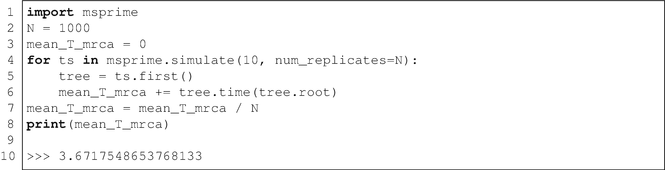In this example we run 1000 independent replicates of the coalescent for a sample of 10 chromosomes, and compute the mean time to the MRCA of the entire sample, i.e., the root of the tree. The value of 3.7 generations in the past we obtain is of course highly unrealistic. However, by default, time is measured in units of 4Ne generations (see the next section for details on how to specify population models and interpret times). It is important to note here that although time is measured in units of generations, this is of course an approximation and we may have fractional values. Internally, during a simulation time is scaled into coalescent units using the Ne parameter and once the simulation is complete, times are scaled back into units of generations before being presented to the user. This removes the burden of such tedious time scaling calculations from the user. We discuss these time scaling issues in more detail in the next section.

The simulate function behaves slightly differently when it is called with the num_replicates argument: rather than returning a single tree sequence, we return an iterator over the individual replicates. This means that we can use the convenient for loop construction to consider each simulation in turn, but without actually storing all these simulations. As a result, we can run millions of replicates using this method without using any extra storage.

When simulating coalescent trees, we are often interested in more than just the mean of the distribution of some statistic. Rather than compute the various summaries by hand (as we have done for the mean in the last example), it is convenient to store the result for each replicate in a NumPy array and analyze the data after the simulations have completed. For example: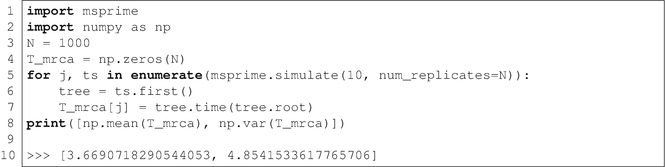Here we simulate 1000 replicates, storing the time to the MRCA for each replicate in the array T_mrca. We use the Python enumerate function to simplify the process of efficiently inserting values into this array, which simply ensures that j is 0 for the first replicate, 1 for the second, and so on. Thus, by the time we finish the loop, the array has been filled with TMRCA values generated under the coalescent. We then use the NumPy library (which has an extensive suite of statistical functions) to compute the mean and variance of this array. This example is idiomatic, and we will use this type of approach throughout. In the interest of brevity, we will omit all further import statements from code chunks.

It is usually more convenient to use the num_replicates argument to perform replication, but there are situations in which it is desirable to specify random seeds manually. For example, if simulations require a long time to run, we may wish to use multiple processes to run these simulations. To ensure that the seeds used in these different processes are unique, it is best to manually specify them. For example,In this example we create a list of 1000 seeds between 1 and 232 − 1 (the range accepted by msprime) randomly. We then use the multiprocessing module to create a worker pool of four processes, and run our different replicates in these subprocesses. The results are then collected together in an array so that we can easily process them. This approach is a straightforward way to utilize modern multi-core processors.

Specifying the same random seed for two different simulations (with the same parameters) ensures that we get precisely the same results from both simulations (at least, on the same computer and with the same software versions). This is very useful when we wish to examine the properties of a specific simulation (for example, when debugging), or if we wish to illustrate a particular example. We will often set the random seed in the examples in this tutorial for this reason.

### 2.2 Population Models

In the previous section the only parameters we supplied to simulate were the sample_size and num_replicates parameters. This allows us to randomly sample trees with a given number of nodes, but, as it leaves the population unspecified, has little connection with biological reality. The most fundamental population parameter is the effective population size, or Ne. This parameter simply rescales time; larger effective population sizes correspond to older coalescence times: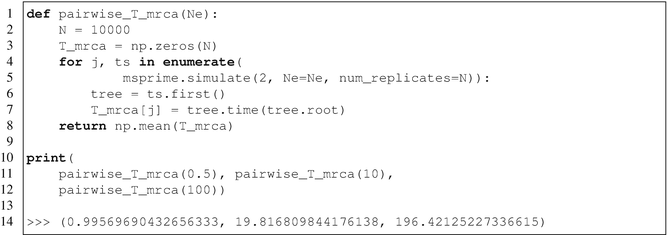Thus, when we specify Ne = 10 we get a mean pairwise coalescence time of about 20 generations, and with Ne = 100, the mean coalescence time is about 200 generations. See ref. 43 for details on the biological interpretation of effective population size.

By default, Ne = 1 in msprime, which is equivalent to measuring time in units of Ne generations. It is very important to note that Ne in msprime is the diploid effective population size, which means that all times are scaled by 2Ne (rather than Ne for a haploid coalescent). Thus, if we wish to compare the results that are given in the literature for a haploid coalescent, then we must set Ne to 1∕2 to compensate. For example, we know that the expected coalescence time for a sample of size 2 is 1, and this is the value we obtain from the pairwise_T_mrca function when we have Ne = 0.5. We will usually assume that we are working in haploid coalescent time units from here on, and so set Ne = 0.5 in most examples. However, when running simulations of a specific organism and/or population, it is substantially more convenient to use an appropriate estimated value for Ne so that times are directly interpretable.

#### 2.2.1 Exponentially Growing/Shrinking Populations

When we provide an Ne parameter, this specifies a fixed effective population size. We can also model populations that are exponentially growing or contracting at some rate over time. Given a population size at the present s and a growth rate α, the size of the population t generations in the past is seαt. (Note again that time and rates are measured in units of generations, not coalescent units.)

In msprime, the initial size and growth rate for a particular population are specified using the PopulationConfiguration object. A list of these objects (describing the different populations; see Section 2.4) are then provided to the simulate function. When providing a list of PopulationConfiguration objects, the Ne parameter to simulate is not required, as the initial_size of the population configurations performs the same task. For example,Here we simulate the pairwise TMRCA for positive, zero, and negative growth rates. When we have a growth rate of zero, we see that we recover the usual result of 1.0 (as our initial size, and hence Ne, is set to 1∕2). When the growth rate is positive, we see that the mean coalescence time is reduced, since the population size is getting smaller as we go backwards in time, resulting in an increased rate of coalescence. Conversely, when we have a negative growth rate, the population is getting larger as we go backwards in time, resulting in a slower coalescence rate. (Care must be taken with negative growth rates, however, as it is possible to specify models in which the MRCA is never reached. In some cases this will lead to an error being raised, but it is also possible that the simulator will keep generating events indefinitely. This is particularly important in simulation based approaches to inference from real data.)

### 2.3 Mutations

We cannot directly observe gene genealogies; rather, we observe mutations in a sample of sequences which ultimately have occurred on genealogical branches. We are therefore very often interested not just in the genealogies generated by the coalescent process, but also in the results of mutational processes imposed on these trees. msprime currently supports simulating mutations under the infinitely many sites model (arbitrarily complex mutations are supported by the underlying data model, however). This is accessed by the mutation_rate parameter to the simulate function. As usual, this rate is the per-generation rate.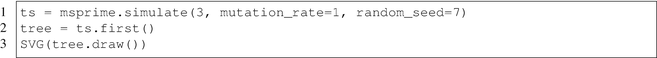The tree produced by this code chunk is shown in Fig. 2. Here we have two mutations, shown by the red squares. Mutations occur above a given node in the tree, and all samples beneath this node will inherit the mutation. The infinite sites mutations used here are simple binary mutations, that is, the ancestral state is 0 and the derived state is 1. One convenient way to access the resulting sample genotypes is to use the genotype_matrix( ) method, which returns an m × n NumPy array, if we have m variable sites and n samples. Thus, if G is the genotype matrix, G[j, k] is the state of the kth sample at the jth site. In our example above, the site 0 has a mutation over node 3, and site 1 has a mutation over node 1, and so we get the following matrix:The genotype matrix gives a convenient way of accessing genotype information, but will consume a great deal of memory for larger simulations. See Section 3.4 for more information on how to access genotype data efficiently.

When comparing simulations to analytic results, it is very important to be aware of the way in which the mutation rates are defined in coalescent theory. For historical reasons, the scaled mutation rate θ is defined as 2Neμ, where μ is the per-generation mutation rate. Since all times and rates are specified in units of generations in msprime, we must divide by a factor of two if we are to compare with analytic predictions. For example, the mean number of segregating sites for a sample of two is θ; to run this in msprime we do the following: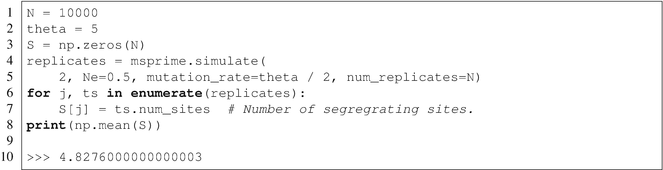Note that here we set the mutation rate to θ∕2 (to cancel out the factor of 2 in the definition of θ) and Ne = 1∕2 (so that time is measured in haploid coalescent time units). Such factor-of-two gymnastics are unfortunately unavoidable in coalescent theory.

### 2.4 Population Structure

Following ms , msprime supports a discrete-deme model of population structure in which d panmictic populations exchange migrants according to the rates defined in an d × d matrix. This approach is very flexible, allowing us to simulate island models (in which all populations exchange migrants at a fixed rate), one- and two-dimensional stepping stone models (where migrants only move to adjacent demes) and other more complex migration patterns. This population structure is declared in msprime via the population_configurations and migration_matrix parameters in the simulate function. The list of population configurations defines the populations; each element of this list must be a PopulationConfiguration instance (each population has independent initial population size and growth rate parameters). The migration matrix is a NumPy array (or list of lists) of per-generation migration rates; m[j, k] defines the fraction of population j that consists of migrants from population k in each generation. (Note that when running simulations on the coalescence scale, i.e. setting Ne = 1∕2, this is equivalent to the number of migrants per deme and generation M[j, k] = 2Nem[j, k].)We create our model by first making a list of two PopulationConfiguration objects. For convenience here, we use the sample_size argument to these objects to state that we wish to have two samples from each population. This results in samples being allocated sequentially to the populations when simulate is called: 0 and 1 are placed in population 0, and samples 2 and 3 are placed in population 1. We then declare our migration matrix, which is asymmetric in this example. Because M[0, 1] = 0.1 and M[1, 0] = 0, forwards in time, individuals can migrate from population 1 to population 0 but not vice versa. This is illustrated in Fig. 3a which shows the tree produced by this simulation. Each node has been colored by its population (red is population 0 and blue population 1). Thus, the leaf nodes 0 and 1 are both from population 0, and 2 and 3 are both from population 2 (as explained above). As we go up the tree, the first event that occurs is 2 and 3 coalescing in population 1, creating node 4. After this, 4 coalesces with node 0, which has at some point before this migrated into deme 1, creating node 5. Node 1 also migrates into deme 1, where it coalesces with 5. Because migration is asymmetric here, the MRCA of the four samples must occur within deme 1.

The exact history of migration events is available if we use the record_migrations option. In the next example, we set up a symmetric island model and track every migration event:Figure 3b shows the tree produced by this code chunk. Here we sample three nodes from population 0, but because there is a lot of migration, the locations of coalescences are quite random. For example, the first coalescence occurs in deme 2 (green), after node 0 has migrated in. To see the details of these migration events, we can examine the “migration records” that are stored by msprime. (These are not stored by default, as they may consume a substantial amount of memory. The record_migrations parameter must be supplied to simulate to turn on this feature.) Migration records store complete information about the time, source, and destination demes and the genomic interval in question. Here we are interested in the total number of migration events experienced by each node:This code produces the plot in Fig. 4. We can see that node 0 experienced very few migration events before it ended up in deme 2, where it coalesced with 4 (which never migrated). Node 2, on the other hand, migrated 30 times before it finally coalesced with 7 in deme 0. Note that there are many more migration events than nodes here, implying that most migration events are not identifiable from a genealogy in real data .

Other forms of migration are also possible between specific demes at specific times. These different demographic events are dealt with in the next section.

### 2.5 Demographic Events

Demographic events allow us to model more complex histories involving changes to the population structure over time, and are specified using the demographic_events parameter to simulate. Each demographic event occurs at a specific time, and the list of events must be supplied in the order they occur (backwards in time). There are a number of different types of demographic event, which we examine in turn.

#### 2.5.1 Migration Rate Change

Migration rate change events allow us to update the migration rate matrix at some point in time. We can either update a single cell in the matrix or all (non-diagonal) entries at the same time.The tree produced by this code chunk is shown in Fig. 5a (in this example and those following we have omitted the code required to draw the tree). The samples 0 and 1, and 2 and 3 coalesce quickly within their own populations. However, because the migration rate between the populations is zero these lineages are isolated and would never coalesce without some change in demography. The migration rate change event happens at time 20, resulting in node 5 migrating to deme 1 soon afterwards. The lineages then coalesce at time 21.4.

#### 2.5.2 Mass Migration

This class of event allows us to move some proportion of the lineages in one deme to another at a particular time. This allows us to model population splits and admixture events. Population splits occur when (backwards in time) all the lineages in one population migrate to another.The tree produced by this code chunk is shown in Fig. 5b. In this case we also have two isolated populations which coalesce down to a single lineage. The population split at time 15 (which, forwards in time produced all the individuals in population 1) results in this lineage migrating back to population 0, where it coalesces with the ancestor of the samples 0, 1, and 2.

Admixture events (i.e., where some fraction of the lineages move to a different deme) are specified in the same way:The tree produced by this code chunk is shown in Fig. 5c. We begin in this example with six lineages sampled in population 0, zero samples in population 1, and with no migration between these populations. At time 0.5, we specify an admixture event where each of the four extant lineages (5, 7, 0, and 6) has a probability of 1/2 of moving to deme 1. Linages 0 and 6 migrate, and subsequently coalesce into node 8. Further back in time, at t = 1.1, another demographic event occurs, changing the migration rate between the demes to 0.1, thereby allowing lineages to move between them. Eventually, all lineages end up in deme 1, where they coalesce into the MRCA at time t = 6.9.

#### 2.5.3 Population Parameter Change

This class of event represents a change in the growth rate or size of a particular population. Since each population has its own individual size and growth rates, we can change these arbitrarily as we go backwards in time. Keeping track of the actual sizes of different populations can be a little challenging, and for this reason msprime provides a DemographyDebugger class.

To illustrate this, we consider a very simple example in which we have a single population experiencing a phase of exponential growth from 750 to 100 generations ago. The size of the population 750 generations ago was 2000, and it grew to 20,000 over the next 650 generations. The size of the population has been stable at this value for the past 100 generations. We encode this model as follows: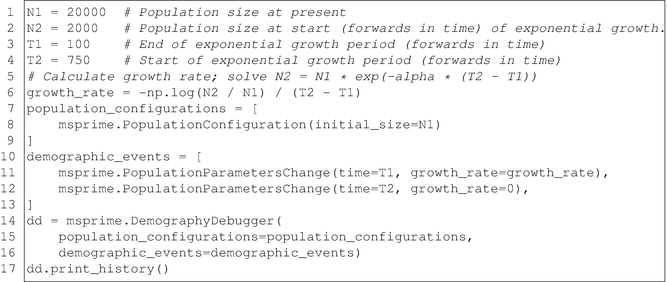It gives the following output:

============================= Epoch: 0 -- 100.0 generations =============================      start     end      growth_rate |     0    -------- --------       -------- | -------- 0 |  2e+04    2e+04               0 |     0 Events @ generation 100.0     - Population parameter change for -1: growth_rate -> 0.0035 ================================= Epoch: 100.0 -- 750.0 generations =================================      start     end      growth_rate |     0    -------- --------       -------- | -------- 0 |  2e+04    2e+03         0.00354 |     0 Events @ generation 750.0    - Population parameter change for -1: growth_rate -> 0 =============================== Epoch: 750.0 -- inf generations ===============================      start     end      growth_rate |     0    -------- --------       -------- | -------- 0 |  2e+03    2e+03               0 |     0

After we set up our model, we use the DemographyDebugger to check our calculations. We see that time has been split into three “epochs.” From the present until 100 generations ago, the population size is constant at 20,000. Then, we have a demographic event that changes the growth rate to 0.0035, which applies over the next epoch (from 100 to 750 generations ago). Over this time, the population grows from 2000 to 20,000 (note that the “start” and “end” of each epoch is looking backwards in time, as we consider epochs starting from the present and moving backwards). At generation 750, another event occurs, setting the growth rate for the population to 0. Then, the population size is constant at 20,000 from generation 750 until the indefinite past.

A more complex example involving a three-population out-of-Africa human model is available in the online documentation.

### 2.6 Ancient Samples

Up to this point we have assumed that all samples are taken at the present time. However, msprime allows us to specify arbitrary sampling times and locations, allowing us to simulate (for example) ancient samples.The tree produced by this code chunk is shown in Fig. 6. All of the trees that we previously considered had leaf nodes at time zero. In this case, the samples 0, 1, and 2 are taken at time 0 in population 0, but node 3 is sampled at time 0.75 in population 1. Note that in this case we used the samples parameter to simulate to specify our samples. This is the most general approach to assigning samples, and allows samples to be assigned to arbitrary populations and at arbitrary times.

### 2.7 Recombination

One of the key innovations of msprime is that it makes simulation of the full coalescent with recombination possible at whole-chromosome scale. Adding recombination to a simulation is simple, requiring very minor changes to the methods given above.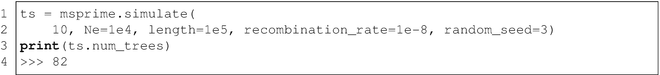In this case, we provide two extra parameters: length, which defines the length of the genomic region to be simulated, and recombination_rate, which defines the rate of recombination per unit of sequence length, per generation. It is often useful to think of both sequence lengths and recombination rates as defined in units of base-pairs. (Note, however, that these are continuous values, so this correspondence should not be taken too literally. Note also that because msprime assumes an infinite sites mutation model the length parameter is not connected to the number of mutational sites. Thus any number of mutations can occur on a given sequence length, depending on the mutation rate specified.) For this example, we defined a sequence length of 100 kb, and a recombination rate of 10−8 per base per generation. The result of this particular simulation is a tree sequence that contains 82 distinct trees. Other replicate simulations with different random seeds will usually result in different numbers of trees.

Up to this point we have focused on simulations that returned a single tree representing the genealogy of a sample. The inclusion of recombination, however, means that there may be more than one tree relating our samples. The TreeSequence object returned by msprime is a very concise and efficient representation of these highly correlated trees. To process the trees, we simply consider them one at a time, using the trees( ) iterator.This code generates the plot in Fig. 7 showing the time of the MRCA of the sample for each tree across the sequence. We find the TMRCA as before, and plot this against the left coordinate of the genomic interval that each tree covers. A full description of tree sequences and the methods for working with them is beyond the scope of this chapter (but see the online documentation for more details).

It is also possible to simulate data with recombination rates varying across the genome (for example, in recombination hotspots). To do this, we first create a RecombinationMap instance that describes the properties of the recombination landscape that we wish to simulate. We then supply this object to simulate using the recombination_map argument. In the following example, we simulate 100 samples using the human chromosome 22 recombination map from the HapMap project . Figure 8 shows the recombination rate and the locations of breakpoints from the simulation, and the density of breakpoints closely follows the recombination rate, as expected.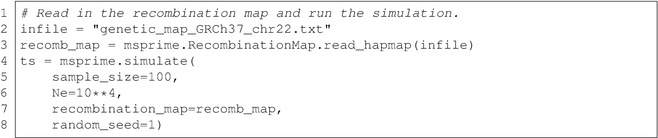Although coordinates are specified in floating point values, msprime uses a discrete loci model when performing simulations. By default, the number of loci is very large (∼232), and the locations of breakpoints are translated back into the coordinate system defined by the recombination map. However, the number of loci is configurable and it is possible to simulate a specific number of discrete loci.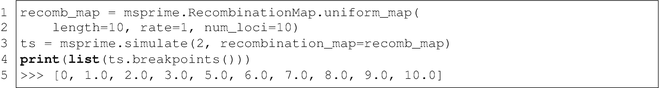Here we simulate the history of two samples in a system with ten loci, each of length 1 with recombination rate of 1 between adjacent loci per generation. In the output, we see that the breakpoints between trees now occur exactly at the integer boundaries between these loci. This shows that we can also simulate models of recombination with discrete loci in msprime, as well as the more standard continuous genome.

## 3 Processing Results

In the previous section we showed how to run simulations in msprime, and how to construct population models and demographic histories. In this section we show how to process the results of simulations. This is not a comprehensive review of the capabilities of the msprime Python API, but concentrates on some useful examples. msprime is specifically designed to enable very large simulations, and the processing methods we demonstrate below are all very efficient. To illustrate this, we consider a simulation of 200,000 samples of ten megabases from a simple two-population model with human-like parameters:This simulation required about 20 s to complete.

### 3.1 Computing MRCAs

We are often interested in finding the most recent common ancestor (MRCA) of a pair (or many pairs) of samples. For example, identity-by-descent (IBD) tracts are defined as contiguous stretches of genome in which the MRCA for a pair of samples is the same. Computing IBD segments for a pair of samples is very straightforward:In this example we create a function ibd_segments that returns a NumPy array of the lengths of IBD segments for a given pair of samples, a and b. It works simply by computing the MRCA for the samples at the left-hand side of the sequence and then, moving rightwards, records a segment each time the MRCA changes. We then plot the distribution of tract lengths for samples 0 and 1 (which are both in population 0), and also the tract lengths for a pair of samples from different populations. The results are shown in Fig. 9. As we might expect, the tract lengths are shorter for the between population pair.

Of course, we would need to sample many such pairs of samples or longer sequences to get a reasonable approximation of the real distribution of block lengths. Because the main cost of this function is the iteration over all the trees in the sequence, it would be more efficient to keep track of the MRCAs for different pairs in a single iteration rather than repeatedly call the above ibd_segments function.

### 3.2 Sample Counts

The msprime API provides an extremely efficient way to count the number of samples that are beneath a particular node in a tree. This can be used, for example, to compute allele frequencies efficiently and is the basis for many of the fast algorithms in the API. As a simple illustration of this technique, consider the following code to compute the number of sites with derived allele frequency less than 1%: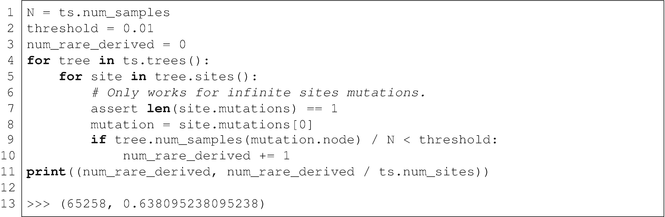In this example we iterate over all the trees in the tree sequence, and then iterate over all the sites in each tree. We find the frequency of the derived allele at each site using the num_samples method, which returns the number of samples subtending a given node. The underlying implementation ensures that this operation requires constant time, and so it is very efficient. We see that such rare alleles are common. (We reiterate that msprime currently generates mutations under the infinitely many sites model so that each mutation occurs at a unique site. Future versions of msprime or other software packages may produce tree sequences with back or recurrent mutations, where this simple approach will not work. To emphasize this point and to ensure that the above code chunk is not accidentally applied in such situations we have included an assert statement. We use asserts in a similar way in later code chunks.)

A powerful feature of this sample-counting approach is that we can perform the same operation over an arbitrary subset of the samples. For example, suppose we wished to count the number of sites that are private to a specific population:This example is very similar, except we provide an extra argument to ts.trees. The tracked_samples argument specifies a list of samples to be tracked, which can be any arbitrary subset of the samples in the simulation. Here we indicate that we are interested in tracking the set of samples within the population in question. Again, we iterate over all trees and over all sites within trees. Then, for each infinite sites mutation we compute two frequencies: the overall number of samples that inherit from the mutation’s node, and the number of tracked samples within the focal population that inherit from this node. If the total count is equal to the within-population count, we know that this mutation is private to the population.

### 3.3 Obtaining Subsets

In some situations it is useful to analyze data for different subsets of the samples separately. This is possible using the simplify method: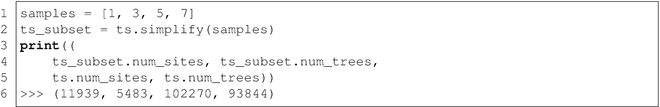Here we extract the tree sequence representing the history of a tiny subset of the original samples, with IDs 1, 3, 5, and 7. The subset tree sequence contains all the genealogical information relevant to the subsamples, but no more. Concretely, both coalescences that are not ancestral to the subsample and coalescences that predate the MRCA of the subsample are excluded. Thus, the number of distinct trees is greatly reduced. By default, we also remove any sites that have no mutations within these subtrees (i.e., those that are fixed for the ancestral state). These can be retained by using the filter_sites=False argument.

Node IDs in the simplified tree sequence are not the same as in the original. The map_nodes argument allows us to obtain the mapping from IDs in the original tree sequence to their equivalent nodes in the new tree sequence.The result of running this code chunk is shown in Fig. 10. Here we draw the first tree in the subset tree sequence, showing the new node IDs along with the IDs from the original tree sequence in parentheses. The number of nodes is greatly reduced from the original.

### 3.4 Processing Variants

While it is nearly always more efficient to work with mutations in terms of their context within the trees, it is sometimes more convenient to work with the allelic states of the samples. This information is obtained in msprime using the variants( ) iterator, which returns a Variant object for each site in the tree sequence. A Variant consists of: (a) a reference to the Site in question; (b) the alleles at this site (the strings representing the actual states); and (c) the genotypes representing the observed state for each sample. The genotypes are encoded in a NumPy array, such that variant.alleles[variant.genotypes[j]] gives the allelic state for sample j. The values in the genotypes array are therefore indexes into the alleles list. The ancestral state at a given site is guaranteed to be the first element in the alleles list, but no other assumptions about ordering of the alleles list should be made.

For biallelic sites, working with genotypes is straightforward as the genotypes array can only contain 0 and 1 values, which correspond to the ancestral and derived states, respectively. The genotypes values are returned as a NumPy array, and so the full NumPy library is available for efficient processing. As an example, we show here how to count the number of sites at which the derived allele is at frequency less than 10%. Using the genotypes in this way is convenient, as complex patterns of back and recurrent mutations can be handled without difficulty.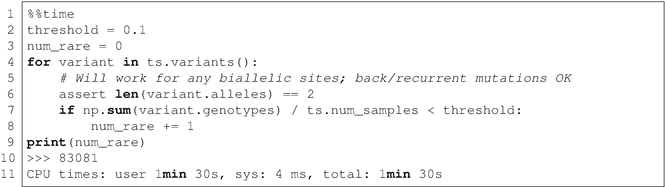This code is straightforward, as we simply iterate over all variants and count the number of one values in the genotypes array. Using the np.sum function, this operation is efficient. Generating all the genotypes for 200,000 samples at 100,000 sites, however, is an expensive operation and the overall calculation takes about 1.5 min to complete.

In the case of infinite sites mutations, we can recast this operation to use the efficient sample counting methods described in Section 3.2. This approach is far more efficient, requiring less than 2 s to compute the same value.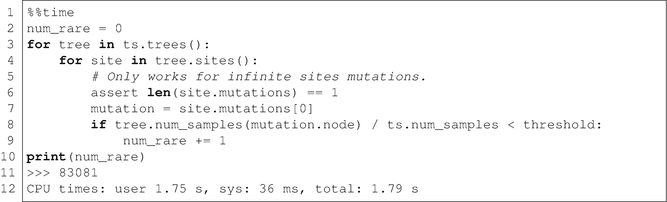### 3.5 Incremental Calculations

A powerful property of the tree sequence representation is that we can efficiently find the differences between adjacent trees. This is very useful when we have some value that we wish to compute that changes in a simple way between trees. The edge_diffs iterator provides us with the information that we need to perform such incremental calculations. Here we use it to keep a running track of the total branch length of our trees, without needing to perform a full traversal each time.This function returns the total branch length value for each tree in the sequence as a NumPy array. It works by keeping track of the total branch length as we proceed from left to right, and storing this value in the output array for each tree. The edge_diffs method returns a list of the edges that are removed for each tree transition (edges_out) and a list of edges that are inserted (edges_in). Computing the current value for the total branch length is then simply a case of subtracting the branch lengths for all outgoing edges and adding the branch lengths for all incoming edges. This is extremely efficient because, after the first tree has been constructed there is at most four incoming and outgoing edges . Thus, each tree transition costs constant time.In contrast, if we compute the total branch length by performing a full traversal for each tree, each tree transition is very costly when we have a large sample size. In this example, computing the array of branch lengths using the incremental approach given here took 8 s. Computing the same array using the tree.total_branch_length for each tree in a straightforward way still had not completed after twenty minutes. (This is because msprime currently implements this operation by a full traversal in Python; in future, this may change to using the algorithm given here.) Full tree traversals of large trees are expensive, and great gains can be made if calculations can be expressed in an incremental manner using edge_diffs.

### 3.6 Exporting Variant Data

If the msprime API doesn’t provide methods to easily calculate the statistics you are interested in, it’s straightforward to export the variant data into other libraries using the genotype_matrix( ) or variants( ) methods. We recommend the excellent scikit-allel  and pylibseq [https://pypi.python.org/pypi/pylibseq] libraries (pylibseq is a Python interface to libsequence ). If you wish to export data to external programs, VCF may be best option, which is supported using the write_vcf method. The simplify method is useful here if you wish to export data from a subset of the simulated samples.

However, it is worth noting that for large sample sizes, exporting genotype data may require a great deal of memory and take some time. One of the advantages of the msprime API is that we do not need to explicitly generate genotypes in order to compute many statistics of interest.

## 4 Validating Analytic Predictions

In this section we show some examples of validating simple analytic predictions from coalescent theory using simulations. The number of segregating sites is the total number of mutations that occurred in the history of the sample (assuming the infinite sites mutation model). Since mutations happen as a Poisson process along the branches of the tree, what we are really interested in is the distribution of the total branch length of the tree. The results in this section are well-known classical results from coalescent theory; this section is intended as a demonstration of how to proceed when comparing analytic results to simulations. We show some idiomatic examples for integrating with the state-of-the-art data analysis packages such as Pandas  and Seaborn . All analytic predictions are taken from .

### 4.1 Total Branch Length and Segregating Sites

The first properties we are interested in are the mean and the variance of the total branch length of coalescent trees. (Note that, as before, we set Ne = 1∕2 to convert between msprime’s diploid time scaling to the haploid time scaling of these analytic results.)We first create an array of the six different n values that we wish to simulate, and then create arrays to hold the results of the simulations. Because we are running 10,000 replicates for each sample size, we allocate arrays to hold 60,000 values. This approach of storing the data in arrays is convenient because it allows us to use Pandas dataframes in an idiomatic fashion. We then iterate over all of our sample sizes and run 10,000 replicates of each. For each simulation, we simply store the sample size value and the total branch length in a Pandas dataframe. This gives us access to many powerful data analysis tools (including the Seaborn library, which we use for visualization here).

After we have created our simulation data, we define our analytic predictions and plot the data.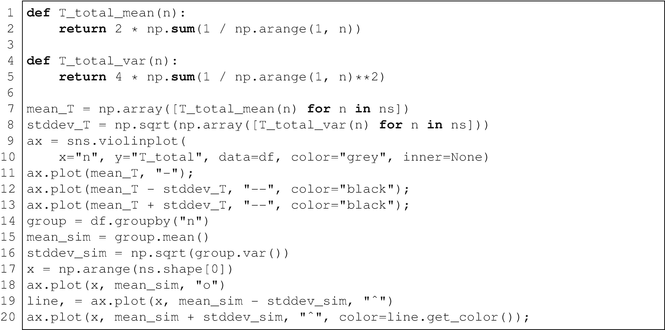The plot in Fig. 11a shows the simulated distribution of the total branch length over replicate simulations (each violin is a distribution for a given sample size). We also show our analytic prediction for the mean and variance of each distribution (the dashed lines show ± 1 standard deviation from the mean). Also shown are the observed means and standard deviations from the simulations, as green circles and red triangles, respectively. We can see that the simulated values match our theoretical predictions for mean and variance very well. We can also see, however, that these one-dimensional summaries of the distribution capture some essential properties but lose some important aspects of the distribution.

Ideally, we wish to capture the full distribution analytically. In the following code chunk we define the analytic prediction for the total branch length distribution, and compare it with the simulated distribution for a sample of size 20. The results are shown in Fig. 11b. We can see an excellent agreement between the smoothed kernel density estimate produced by Seaborn and the theoretical prediction.Since we cannot directly observe branch lengths, we are usually more interested in mutations when working with data. The mutation process is intimately related to the distribution of branch lengths, since mutations occur randomly along tree branches. One simple summary of the mutational process is the total number of segregating sites, that is, the number of sites at which we observe variation. We can obtain this very easily from simulations simply by specifying a mutation rate parameter. (Note again that we set Ne = 1∕2 and our mutation rate = θ∕2 in order to convert to msprime’s time scales.)Here we take 1000 replicate simulations, store the number of infinite sites mutations for each, and plot this distribution in Fig. 12a. Also plotted is the analytic prediction, which again provides an excellent fit.

### 4.2 Recombination

In the previous section we saw how to run simulations to generate trees under the assumptions of the single-locus coalescent and compare these with analytic predictions. This assumes that our data is not affected by recombination, which is often unrealistic. Here we show how to compute empirical distributions of equivalent quantities, and compare these with classical results from the literature. Since analytic results for many quantities are generally unknown for the case of recombination along a linear sequence, we limit ourselves to the pairwise samples.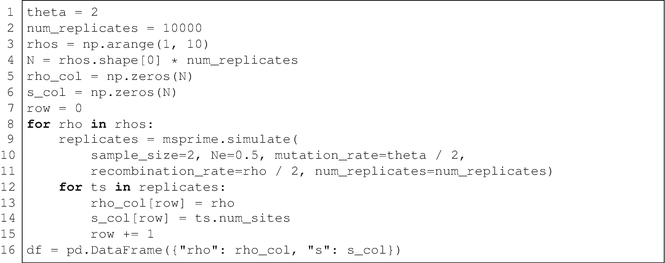In this code chunk we again run 104 replicate simulations for a range of input parameters, and store the results in a Pandas data frame. We are interested in the effects of recombination rate in this example, and so the parameter that we vary is the scaled recombination rate ρ (noting, again, that we set Ne = 1∕2 and recombination_rate = ρ∕2 to convert to msprime’s time scales).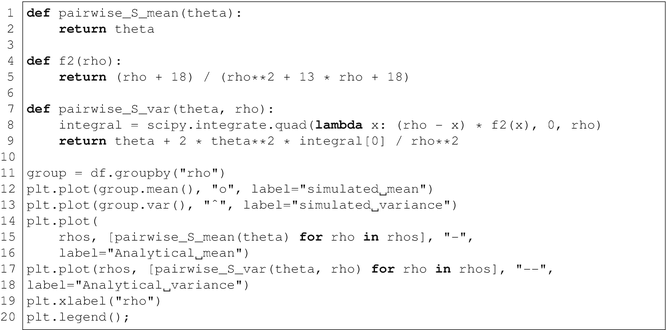After defining our analytic predictions for the mean and variance of the number of segregating sites, we then plot the observed and predicted values in Fig. 12b. Comparing the simulated results to analytic predictions we see excellent agreement. The mean number of segregating sites is not affected by recombination, but recombination does substantially reduce the variance.

## 5 Example Inference Scheme

The analytical challenges of deriving likelihood functions even under highly idealized models of population structure and history have led to the development of likelihood-free inference methods, in particular Approximate Bayesian Computation (ABC) . ABC approximates the posterior distribution of model parameters by drawing from simulations. Because of its flexibility ABC has become a standard inference tool in statistical population genetics (see ref. 7, for a review). We will demonstrate how msprime can be used to set up an ABC inference by means of a simple toy example. We stress that this is meant as an illustration rather than an inference tool for practical use. However, given the flexibility of msprime, it should be relatively straightforward to implement more a realistic framework focused on specific inference applications.

We assume that data for 200 loci or sequence blocks (these could be RAD loci in practice) for a single diploid individual have been generated from each of two populations. We would like to infer the amount of gene flow between the two populations. For the sake of simplicity, we will assume the simplest possible model of population structure; that is, two populations, of the same effective size exchanging migrants at a constant rate of m migrants per generation.

The function run_sims simulates a dataset consisting of a specified number of loci (num_loci) given a migration rate M. We generate a single dataset of 50 loci assuming a migration rate M = 0.3 migrants per generation, which we will use as a (pseudo)observed dataset in the ABC implementation.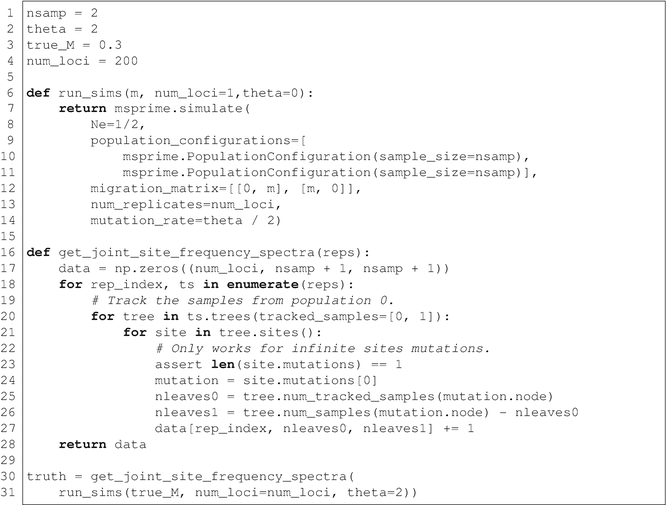The run_sims function returns an iterator with the complete tree sequence and mutational information of each locus. We use the function get_joint_site_frequency_spectra to summarize the polymorphism information as the joint site frequency spectrum (jSFS) of each locus, i.e. the blockwise site frequency spectrum or bSFS [sensu 28]. Note that higher level population genetic summaries, e.g. pairwise measures of divergence and diversity such as DXY  and FST  or multi-population F statistics [8, 34] which are often used in ABC inference are just further (and lossy) summaries of the jSFS.

Since msprime simulates rooted trees, the columns and rows of the unfolded jSFS correspond to the frequency of derived mutations in each population and the entries of the jSFS are simply mutation counts. For example, for the first locus we have: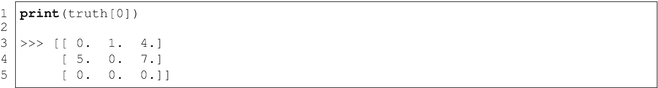One could base inference on the bSFS [4, 28], but we will for the sake of simplicity use a simpler (and lossy) summary of the data: the average jSFS across loci. For analyses based on SNPs, it is convenient to normalize the jSFS by the total number of mutations: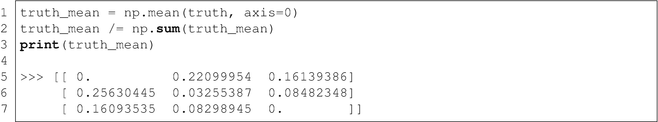To illustrate a simple ABC inference, we will focus on a single parameter of interest, the migration rate M. ABC measures the fit of data simulated under the prior to the observed data via a vector of summary statistics. We will use the jSFS as a summary statistic and approximate the jSFS for each M value as the mean length of genealogical branches across 100 simulation replicates (num_reps). Below we draw 10,000 M values from the prior and use the functions run_sims and approx_jSFS to approximate the jSFS for replicate. We assume an exponential distribution, a common choice of prior .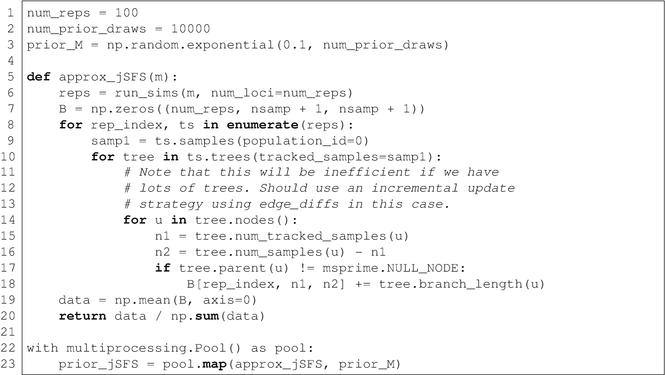Here we run 100 simulation replicates for each of the 10,000 m values drawn from the prior, giving a total of one million individual simulations. We use the multiprocessing module to distribute these computations over the available CPU cores. Once this has completed, we compute the Euclidean distance between the estimated jSFS for each draw from the prior (prior_jSFS) and the jSFS in the (pseudo)observed data (truth_mean):In its simplest form, ABC approximates the posterior by sampling from the simulated data via an acceptance threshold. Here we approximate the posterior distribution of m using the 5% of simulation replicates that most closely match the average jSFS of the observed data. Figure 13a shows that the posterior distribution (shown in green) is centered around m = 0.25.The mean and the 95% approximate posterior credible interval for m are:Although the true value of m = 0.3 is contained within the 95% credible interval, the posterior distribution is clearly downwardly biased. This bias is in fact expected given that our prior is also strongly biased towards low m. We can check the effect the acceptance threshold on the inference and get a sense of the expected information about m using a cross-validation procedure: we repeat the inference on pseudo-observed data sets (PODS) simulated under a known truth. Since we can re-use the same set of replicates simulated under the prior for inference, such cross-validation is computationally efficient.

Figure 13b shows the mean and the root mean square error (RMSE) of m estimates (across 100 PODS) against the acceptance threshold and confirms that both the downward bias in m estimates and the associated RMSE increase with larger acceptance thresholds. While this toy example illustrates the principle of ABC inference, sampling only a small fraction of simulations generated under the prior is clearly computationally inefficient and more efficient sampling strategies for ABC inference have been developed . In practice, we are generally interested in fitting parameter-rich models and it would be straightforward to implement ABC inference for complex model of population structure and demography in msprime.

## 6 Discussion

In this chapter we have focused on the usage of msprime as a coalescent simulator, and illustrated its flexibility through concrete examples. While many examples discuss how to create and run the simulations themselves, others are concerned with how we analyze the output of these simulations. We have shown particularly in Section 3 that these methods can be very efficient, allowing us to easily analyze chromosome scale data for hundreds of thousands of samples. The data structures and APIs used in msprime are currently being developed to increase their generality and applicability. Recent work [11, 24] has shown that forward-time simulations can also benefit from these methods. By recording all genealogical information for the simulated population in the form of a succinct tree sequence, we avoid the need to generate and carry forward neutral mutations; by definition, they do not affect the genealogies, and can therefore be placed on them afterwards. Not only does this provide us with much more complete information about the forward-time simulation, it also leads to substantially faster running times (up to 50× faster, in the simulations performed). Through the use of a well-documented interchange API and thoroughly specified data formats, forward-time simulators can output data that is compatible with the msprime API, and precisely the same techniques described here can be used to analyze the results. Thus, code written to analyze coalescent simulations can equally be applied to analyze forwards simulations.

There is currently a great deal of activity from a growing community around msprime. We plan to separate the tree sequence processing code from the simulator and create a library, provisionally known as tskit. This standalone library (C and Python interfaces are planned) will greatly facilitate integration with forwards-time simulators, allowing them to easily offload tree sequence processing to tskit. Algorithms for efficiently calculating statistics using the incremental techniques outlined in Section 3.5 are in development, and promise to be significantly more efficient than the state of the art. Also in development are methods to estimate the tree sequence data structure from real data, which would allow us to use these efficient algorithms on observed as well as simulated data. New features are being added to the msprime simulator also, with support for a discrete time Wright-Fisher model and a family of multiple-merger coalescent models in development. We hope that in the coming years a diverse ecosystem of tools and applications using these APIs and data structures will emerge.

### Online Resources

 Jupyter notebook https://github.com/StatisticalPopulationGenomics/msprime Documentation https://msprime.readthedocs.io/en/stable/ GitHub https://github.com/tskit-dev/msprime Mailing list https://groups.google.com/forum/#!forum/msprime-users

## Change history

• ### 27 February 2021

Chapter 2, “Processing and Analyzing Multiple Genomes Alignments with MafFilter,” was previously published without including the Electronic Supplementary Material. This has now been included in the revised version of this book.

## References

1. Arenas M (2012) Simulation of molecular data under diverse evolutionary scenarios. PLoS Comput Biol 8(5):e1002495

2. Beaumont MA, Zhang W, Balding DJ (2002) Approximate Bayesian computation in population genetics. Genetics 162:2025–2026

3. Becquet C, Przeworski M (2007) A new approach to estimate parameters of speciation models with application to apes. Genome Res 17(10):1505–1519

4. Beeravolu Reddy C, Hickerson MJ, Frantz LAF, Lohse K (2017) Blockwise site frequency spectra for inferring complex population histories and recombination, bioRxiv. https://doi.org/10.1101/077958

5. Carvajal-Rodríguez A (2008) Simulation of genomes: a review. Curr Genomics 9(3):155–159

6. Cornuet JM, Santos F, Beaumont MA, Robert CP, Marin JM, Balding DJ, Guillemaud T, Estoup A (2008) Inferring population history with DIY ABC: a user-friendly approach to approximate Bayesian computation. Bioinformatics 24(23):2713–2719

7. Csilléry K, Blum M, Gaggiotti OE, François O (2010) Approximate Bayesian computation (ABC) in practice. Trends Eco Evol 25(7):410–418

8. Durand EY, Patterson N, Reich D, Slatkin M (2011) Testing for ancient admixture between closely related populations. Mol Biol Evol 28(8):2239–2252

9. Excoffier L, Dupanloup I, Huerta-Sánchez E, Sousa VC, Foll M (2013) Robust demographic inference from genomic and SNP data. PLoS Genet 9(10):e1003905

10. Gutenkunst RN, Hernandez RD, Williamson SH, Bustamante CD (2009) Inferring the joint demographic history of multiple populations from multidimensional SNP frequency data. PLoS Genet 5(10):e1000695

11. Haller BC, Galloway J, Kelleher J, Messer PW, Ralph PL (2018) Tree-sequence recording in SLiM opens new horizons for forward-time simulation of whole genomes, bioRxiv. https://doi.org/10.1101/407783. https://www.biorxiv.org/content/early/2018/09/04/407783

12. Harris K, Nielsen R (2013) Inferring demographic history from a spectrum of shared haplotype lengths. PLoS Genet 9(6):e1003521

13. Hey J, Nielsen R (2004) Multilocus methods for estimating population sizes, migration rates and divergence time, with applications to the divergence of Drosophila pseudoobscura and D. persimilis. Genetics 167(2):747–760

14. Hoban S, Bertorelle G, Gaggiotti OE (2012) Computer simulations: tools for population and evolutionary genetics. Nat Rev Genet 13(2):110

15. Hudson RR (1983) Testing the constant-rate neutral allele model with protein sequence data. Evolution 37(1):203–217

16. Hudson RR (1990) Gene genealogies and the coalescent process. Oxf Surv Evol Biol 7:1–44

17. Hudson RR (2002) Generating samples under a Wright-Fisher neutral model of genetic variation. Bioinformatics 18(2):337–338

18. Hunter JD (2007) Matplotlib: a 2d graphics environment. Comput Sci Eng 9(3):90–95

19. International HapMap Consortium (2003) The international HapMap project. Nature 426(6968):789

20. Jones E, Oliphant T, Peterson P, et al (2018) SciPy: open source scientific tools for Python (2001–2018). http://www.scipy.org/ [Online; Accessed 30 Jan 2018]

21. Kelleher J, Barton NH, Etheridge AM (2013) Coalescent simulation in continuous space. Bioinformatics 29(7):955–956

22. Kelleher J, Etheridge A, Barton N (2014) Coalescent simulation in continuous space: algorithms for large neighbourhood size. Theor Popul Biol 95:13–23

23. Kelleher J, Etheridge AM, McVean G (2016) Efficient coalescent simulation and genealogical analysis for large sample sizes. PLoS Comput Biol 12(5):e1004842

24. Kelleher J, Thornton K, Ashander J, Ralph P (2018) Efficient pedigree recording for fast population genetics simulation. PLoS Comput Biol 14(11):e1006581

25. Kingman JFC (1982) The coalescent. Stoch Processes Appl 13(3):235–248

26. Li H, Durbin R (2011) Inference of human population history from individual whole-genome sequences. Nature 475:493–496

27. Liu Y, Athanasiadis G, Weale ME (2008) A survey of genetic simulation software for population and epidemiological studies. Hum Genomics 3(1):79

28. Lohse K, Chmelik M, Martin SH, Barton NH (2016) Efficient strategies for calculating blockwise likelihoods under the coalescent. Genetics 202(2):775–786

29. Martin AR, Gignoux CR, Walters RK, Wojcik GL, Neale BM, Gravel S, Daly MJ, Bustamante CD, Kenny EE (2017) Human demographic history impacts genetic risk prediction across diverse populations. Am J Hum Genet 100(4):635–649

30. McKinney W, et al (2010) Data structures for statistical computing in python. In: Proceedings of the 9th Python in science conference, Austin, TX, vol 445, pp 51–56

31. McVean GAT, Cardin NJ (2005) Approximating the coalescent with recombination. Philos Trans R Soc Lond B Biol Sci 360(1459):1387–1393

32. Miles A, Harding N (2017) scikit-allel. https://doi.org/10.5281/zenodo.822784

33. Nei M (1972) Genetic distance between populations. Am Nat 106(949):283–292

34. Patterson N, Moorjani P, Luo Y, Mallick S, Rohland N, Zhan Y, Genschoreck T, Webster T, Reich D (2012) Ancient admixture in human history. Genetics 192(3):1065–1093

35. Pérez F, Granger BE (2007) Ipython: a system for interactive scientific computing. Comput Sci Eng 9(3):21–29

36. Rasmussen MD, Hubisz MJ, Gronau I, Siepel A (2014) Genome-wide inference of ancestral recombination graphs. PLoS Genet 10(5):e1004342

37. Schiffels S, Durbin R (2014) Inferring human population size and separation history from multiple genome sequences. Nat Genet 46:919–925

38. Sousa VC, Grelaud A, Hey J (2011) On the nonidentifiability of migration time estimates in isolation with migration models. Mol Ecol 20(19):3956–3962

39. Staab PR, Zhu S, Metzler D, Lunter G (2014) scrm: efficiently simulating long sequences using the approximated coalescent with recombination. Bioinformatics 31(10):1680–1682

40. Tajima F (1983) Evolutionary relationship of DNA sequences in finite populations. Genetics 105(2):437–460

41. Thornton K (2003) Libsequence: a C++ class library for evolutionary genetic analysis. Bioinformatics (Oxf, Engl) 19(17):2325–2327

42. van der Walt S, Colbert SC, Varoquaux G (2011) The NumPy array: a structure for efficient numerical computation. Comput Sci Eng 13(2):22–30

43. Wakeley J (2008) Coalescent theory: an introduction. Roberts and Company, Englewood

44. Waskom M, Botvinnik O, O’Kane D, Hobson P, Lukauskas S, Gemperline DC, Augspurger T, Halchenko Y, Cole JB, Warmenhoven J, de Ruiter J, Pye C, Hoyer S, Vanderplas J, Villalba S, Kunter G, Quintero E, Bachant P, Martin M, Meyer K, Miles A, Ram Y, Yarkoni T, Williams ML, Evans C, Fitzgerald C, Brian, Fonnesbeck C, Lee A, Qalieh A (2017) mwaskom/seaborn: v0.8.1 (September 2017). https://doi.org/10.5281/zenodo.883859

45. Wright S (1950) Genetical structure of populations. Nature 166:247–249

46. Yuan X, Miller DJ, Zhang J, Herrington D, Wang Y (2012) An overview of population genetic data simulation. J Comput Biol 19(1):42–54

## Acknowledgements

We would like to thank Simon Aeschbacher for comments on the ABC inference example, and to thank Yan Wong, Joseph Marcus, and Julien Dutheil for detailed and insightful feedback. JK is supported by Wellcome Trust grant 100956/Z/13/Z to Gil McVean. KL is supported by an Independent Research fellowship from the Natural Environment Research Council (NE/L011522/1).

## Author information

Authors

### Corresponding author

Correspondence to Jerome Kelleher .

## Rights and permissions

Open Access This chapter is licensed under the terms of the Creative Commons Attribution 4.0 International License (http://creativecommons.org/licenses/by/4.0/), which permits use, sharing, adaptation, distribution and reproduction in any medium or format, as long as you give appropriate credit to the original author(s) and the source, provide a link to the Creative Commons license and indicate if changes were made.

The images or other third party material in this chapter are included in the chapter's Creative Commons license, unless indicated otherwise in a credit line to the material. If material is not included in the chapter's Creative Commons license and your intended use is not permitted by statutory regulation or exceeds the permitted use, you will need to obtain permission directly from the copyright holder.

Reprints and Permissions